MATH 3181 - 001 FALL 1996

Axioms and Definitions
for Plane Geometry

The Euclidean plane will be denoted by E2 and the hyperbolic plane will be H2. If we are referring to either of them, without specificity, we will use the notation P.

The plane is a set of points (undefined terms) and a line is a subset of the plane subject to the axioms that follow. If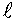is a line and A is a point in that subset, we say that A lies onorpasses through A.

Axiom 1: If A and B are distinct points, then there is one and only one line that passes through them both.

Axiom 2: There is a function |PQ| defined for all pairs of points P,Q such thatand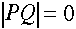if and only if P=Q. This function satisfies the following properties:for all triples of points A, B,and C.

This axiom makes the plane into a metric space and |PQ| is called the distance between P and Q.

Axiom 3: For each line , there is a one-to-one function,, such that if A and B are any points on, then |AB| = |x(A) - x(B)|.

This mapping is not unique because we can use this same mapping and add a constant. On the other hand, it does say that each of our lines is isometric to the set of real numbers and, hence, are all isometric to one another.

This mappingis a coordinatization of the line. That is, it defines a coordinate system on the line. There is a point that corresponds to 0 and there are points that correspond to the positive real numbers and points that correspond to the negative real numbers. If A and B are points of, then the segment AB ofis the set of points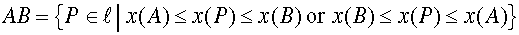and the number |AB| is its length. The points A and B are its endpoints. When we have a coordinatization, we say that the lineis a directed line. If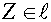, the set of points A such thatis a raywith Z as its origin or initial point. The set of points A such thatis called the opposite ray.

Axiom 4: Ifis any line, there are two corresponding subsets HP1 and HP2, called half-planes, such that the sets , HP1, and HP2 are disjoint, and the union of these sets andis all of P, such that:

1. If P and Q are in the same half-plane, the segment PQ contains no point of, while
2. if P and Q are in opposite half-planes, the segment PQ contains exactly one point of.

We say that P and Q are on the same side of or on opposite sides of.

Two rays with a common origin, A, constitute an angle with A as its vertex. Other authors insist that the two rays are not opposite rays, but no such restriction is made here. If the two rays are not the same ray nor opposite rays, then the interior of the angle is the set of all points on the same side ofas C and on the same side ofas B. The interior of the triangle ABC is the intersection of the interiors of its three angles. If two lines intersect at the point A then the two angles that share a common side are called supplementary angles and the two angles that do not share a common side are called vertical angles.

Axiom 5: (Measures of angles) For each anglethere is a number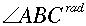in the interval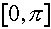, called the (radian) measure of the angle such that

1. If the two rays comprising the angle are the same ray, the measure is 0; if they are opposite rays, the measure is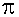;
2. the sum of the measures of an angle and its supplement is;
3. ifis in the interior ofthen;
4. if a ray from the point A lies in a line then in each half-plane bounded by, the set of rays from A is in a one-to-one correspondence with the set of real numbers in;
5. if the ray in part (d) contains the point P, then the angle measure depends continuously on P.

Two segments with the equal lengths are called congruent segments and two angles with equal measures are called congruent angles. A right angle is an angle that is congruent with its supplement.

Given three non-collinear points A, B, and C the triangle ABC is the union of the three segments AB, BC, and AC. The points A, B, and C are called the vertices, the segments are called the sides and the three angles defined by these segments are called its angles.

Axiom 6: (SAS Axiom) If two sides and the included angle of a first triangle are congruent respectively to two sides and the included angle of a second triangle, then the triangles are congruent. That is, the remaining sides are congruent and the remaining angles are congruent in pairs.

Parallel Axiom: There are two distinct choices for the Parallel Axiom:

(Euclidean Parallel Axiom) Given any line and any point not on that line, there is only one line through the given point that never intersects the given line.

(Hyperbolic Parallel Axiom) There exists a line and a point not on that line such that there are at least two lines through that point that do not intersect the given line.This site is best viewed with Netscape Navigator.Back to the My Home Page